American Journal of Theoretical and Applied Statistics
Volume 5, Issue 5, September 2016, Pages: 326-333

Poisson Inverse Gaussian (PIG) Model for Infectious Disease Count Data

Vincent Moshi Ouma1, *, Samuel Musili Mwalili2, Anthony Wanjoya Kiberia2

1Applied Statistics, Department of Statistics and Actuarial Sciences, College of Pure and Applied Sciences, Jomo Kenyatta University of Agriculture and Technology, Nairobi, Kenya

2Department of Statistics and Actuarial Sciences, College of Pure and Applied Sciences, Jomo Kenyatta University of Agriculture and Technology, Nairobi, Kenya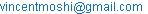(V. M. Ouma)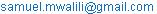(S. M. Mwalili)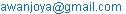(A. W. Kiberia)

Vincent Moshi Ouma, Samuel Musili Mwalili, Anthony Wanjoya Kiberia. Poisson Inverse Gaussian (PIG) Model for Infectious Disease Count Data. American Journal of Theoretical and Applied Statistics. Vol. 5, No. 5, 2016, pp. 326-333. doi: 10.11648/j.ajtas.20160505.22

Received: September 9, 2016; Accepted: September 21, 2016; Published: October 10, 2016

Abstract: Traditionally, statistical models provide a general basis for analysis of infectious disease count data with its unique characteristics such as low disease counts, underreporting, reporting delays, seasonality, past outbreaks and lack of a number of susceptible. Through this approach, statistical models have provided a popular means of estimating safety performance of various health elements. Predictions relating to infectious disease outbreaks by use of statistical models have been based on Poisson modeling framework and Negative Binomial (NB) modeling framework in the case of overdispersion within the count data. Recent studies have proved that the Poisson- Inverse Gaussian (PIG) model can be used to analyze count data that is highly overdispersed which cannot be effectively analyzed by the traditional Negative Binomial model. A PIG model with fixed/varying dispersion parameters is fitted to two infectious disease datasets and its performance in terms of goodness-of-fit and future outbreak predictions of infectious disease is compared to that of the traditional NB model.

Keywords: Mixed Models, Poisson-Inverse Gaussian Distribution, Negative Binomial Distribution, Infectious Disease

1. Introduction

An everlasting fight for humans to cab the virulent of various viruses has been ongoing since time in memorial. This has been done by use of statistical models. Since data from infectious disease are count data, most frequently researchers and scholars alike have tended to favor the use of Poisson distribution as the base distribution for the counts and in addition, zero inflation to counter the excessive zeros that are prominent in most infectious disease data. The NB distribution, a mixture of Poisson and Gamma distributions, has been applied to account for overdispersion usually encountered in most infectious diseases count data . But with the restrictive nature of the Poisson models (equal dispersion), and the NB models less flexibility approach in handling highly dispersed data, they pose a great challenge in modeling infectious disease count data with the corresponding characteristics. A few extensions within the model to allow for higher dispersion is needed. A few studies suggest the PIG model as an alternative to the NB model for modeling count data especially those with longer tails and larger kurtosis  . Further extensions of these in recent past have led to the development of mixed models that have the capabilities of handling effectively count data with unique characteristics common to infectious diseases .

This paper will involve the use of a mixed Poisson- Inverse Gaussian distribution in modeling count data from infectious diseases by using a parameter -and observation-driven model for infectious disease and compare its performance to that of the traditional Negative Binomial distribution.

2. Poisson Distribution

The basic of all regression models for count data is the Poisson regression model  expressed as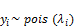(1)

Where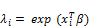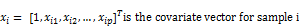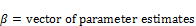Assuming an equal dispersion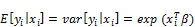(2)

3. Negative Binomial Distribution

For overdispersed count data, the Poisson model can be modified asWhere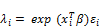(3)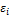Is the non-negative (e.g. gamma, lognormal etc) multiplicative random-effect term that assists in modeling heterogeneity .

Incorporating the use of the law of total expectation and the law of total variance it is evident that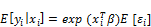(4)

and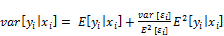(5)

This suggests that for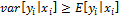we obtain a regression model for overdispersed counts .

Even though the Poisson models have been extensively applied to model count events , the assumption that the mean and the variance of the count data are equal is rather too restrictive and rarely does occur in observational data. Furthermore, when observed data involves excessive zero counts, overdispersion arises hence may lead to underestimating the variance of the estimated parameter, leading to the wrong conclusion . Due to overdispersion NB model was presented as a good alternative to the Poisson model. This is because the NB model allows the extra variation within the data to be captured by adding a randomly distributed error term, that is based on the Gamma distribution, that is, the structure of the NB regression model is constructed by allowing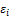have a prior gamma;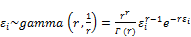(6)

Where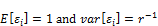Thus the NB distribution is parameterized by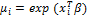and an inverse parameter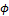(reciprocal of r) as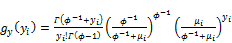(7)

Hence, we have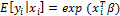and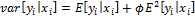.

Since the inception of the NB model, it has been widely favored to model count data from infectious disease due to the flexibility and less strictness on the gamma part    . In the recent past, other statistical regression models for analyzing count data exhibiting overdispersion and excess zero counts have been proposed and show potential alternatives for the traditionally NB regression model. They include.

4. Review of Some Count Mixed Models

4.1. The Zero-Inflated and the Hurdle Models

The Zero-inflated and Hurdle models are both known to handle count data with excessive zeros in the observed data. The Zero-inflated model assumes that the zero observations have two distinct different origins, that is, structural and sampling (chance). On the other hand, Hurdle models assume all zero data are from structural source with the nonzero data having sampling origin with either truncated Poisson or truncated NB distributions .

For a further review of Zero-inflated and Hurdle models, check Hu et al .

4.2. Lognormal-Poisson Regression Model

Agresti  constructs a lognormal-Poisson regression model by inputting a lognormal prior onas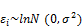(8)

Where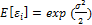and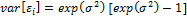Hence we have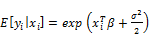(9)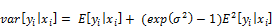(10)

4.3. The Log-normal and Gamma Mixed NB (LGNB) Regression Model

Since Bayesian analysis of counts is limited in that, their lack an efficient inference as to the conjugate prior for the regression coefficient β is unknown under the Poisson and NB likelihoods  and also the conjugate prior of the NB dispersion parameter is unknown. The Log-normal and gamma mixed NB regression model addresses these issues by inputting a lognormal prior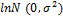as multiplicative random effect term onand a gamma prior on the dispersion parameter r.

Let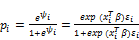(11)

and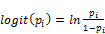(12)

Then the LGBN model is expressed as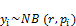(13)

Where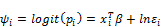(14)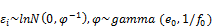(15)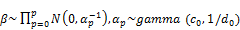(16)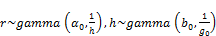(17)

and

ψ can be modeled as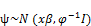(18)

where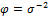and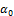,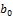,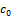,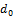,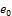,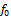and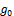are gamma hyperparameters.

4.4. The Negative Binomial-Lindley (NB-L) Model

Even though models mentioned above are considered to provide a better fit than the traditional NB model, many of the models show a deficiency in analyzing datasets with a large number of zeros and are highly skewed . Geedipally  introduced the Negative Binomial-Lindley (NB-L) model in modeling count data characterized by a large number of zeros.

The NB-L distribution is a mixture of NB and Lindley’s distributions resulting into a mixed distribution with a thick tail and useful for data containing a large number of zeros. Zamani and Ismail , gives the pmf of the NB-L distribution as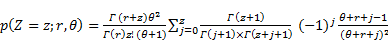(19)

Where

r is the shape parameter of NB-L distribution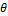in combination with shape parameter r dictates the mean and variance of the NB-L distribution.

The mean of the NB-L (r,) is given as;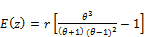(20)

Lord et al.  demonstrated that the NB-L model provides a better statistical performance than the ZINB and it is more theoretically sound. However, the only disadvantage of the NB-L model is that its likelihood function does not have a closed form hence the parameter estimation based on McMC chain requires intensive computation time .

4.5. The Sichel (SI) Model

Hauer  in his research points out that although the gamma assumption for theis adequate for various datasets, it is not a proof thatare indeed gamma distributed. He notes that there is a need to explore other mixed-Poisson models. Zou et al.  introduced the Sichel (SI) distribution for modeling highly dispersed count data. The SI distribution is a compound of Poisson distribution that mixes Poisson distribution with generalized inverse Gaussian distribution.

The Sichel distribution SI (μ,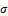, ν) has the following structure;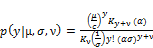(21)

Where y, μ,, ν are response variable, mean, scale parameter, shape parameter respectively and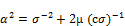(22)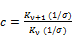(23)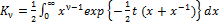is the Bessel function.

For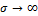and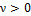, the SI distribution reduces to a NB distribution.

5. Poisson Inverse Gaussian (PIG) Distribution

The PIG distribution is a special case of the SI distribution in which the shape parameter is set to be -0.5. Thus it is characterized by only two parameters. Its likelihood function is easily obtainable and has a closed form, indicating the estimation of parameters as quite simple and almost takes no time .

As demonstrated by Zha et al. , the overall PIG distribution denoted as PIG (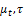), is given by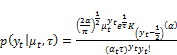(24)

Where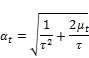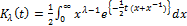is the Bessel function of third kind

The mean and variance of the PIG distribution are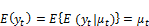(25)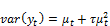(26)

6. Methodology

The functional form of the model is given by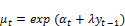(27)

where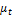is the mean number of counts per week,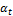endemic component, and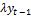endemic component.

The form of the dispersion parameters is nonlinear since it provides more flexibility to capture the variance of the data .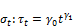(28)

Where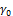and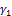are estimated coefficients.

The endemic component is defined as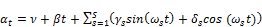(29)

Where s is the number of harmonics to include and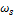is the Fourier frequencies i.e.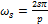where p is the base frequencies (week in our case).

The epidemic component is considered as observations driven process through parameter λ. For λ= 0, the model reduces to a parameter-driven formulation with no epidemic incidence and for 0 <λ < 1, the model displays occasional epidemic outbreaks.

6.1. Estimation of Model Parameters

An advantage of the proposed model is that its framework is easily estimated by Maximum likelihood method . A further advantage is that the likelihood function of the PIG distribution has a closed form hence, enabling the regression parameter to be easily obtained through MLE method . As proposed by Dean et al. , the probability generating function (PGF) for PIG (μ,) is given by;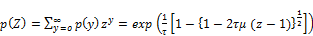(30)

Calculating recursively the probabilities, we have;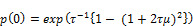(31)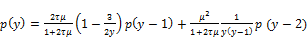(32)

For y=2, 3, …..

For a random sample of observations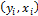, the log likelihood function can be derived from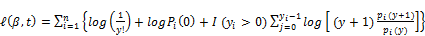(33)

6.2. Data Description

Two datasets used in the analysis are weekly salmonella Hadar observed cases in Germany from the years 2001 to 2006 and weekly Salmonella Agona observed cases in the UK from the years 1990 to 1995. The two dataset are provided within the Surveillance package in R.

The statistics involved in describing the data is skewness, kurtosis, and variance to mean ratio.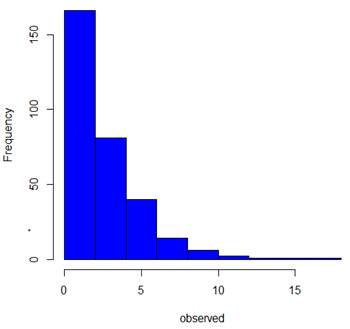Figure 1. Salmonella agona data distribution.

6.2.1. Salmonella Agona Data

The Salmonella Agona data set included 312 observed cases of the disease within the UK over a period of six years from 1990 to 1995. The distribution of the data is as shown in figure 1. From the distribution, it is evident that the data has a long tail and rightly skewed with a skewness coefficient of 1.68 indicating high skewness . The variance over mean ratio is 2.436. This information suggests that the data is overdispersed. 16% out of the 312 cases reported zero counts.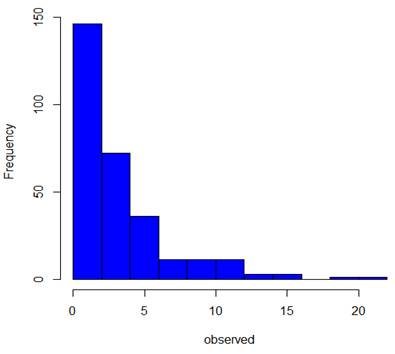Figure 2. Salmonella hadar data distribution.

The Salmonella Hadar data set included 295 observed cases of the disease within Germany over a period of six years from 2001 to 2006. The distribution of the data is as shown in figure 2. From the distribution, it is evident that the data has a long tail and rightly skewed with a skewness coefficient of 1.85 indicating high skewness. The variance over mean ratio is 3.45. This information suggests that the data is overdispersed. 14% out of the 295 cases reported zero counts.

7. Results and Discussion

7.1. Goodness of Fit

The goodness-of-fit test was based on the values of the global deviance, Akaike Information Criterion (AIC) and Bayesian Information Criterion (BIC). Small values of this meant that the respective model provided the best fit for the data.

Table 1 and Table 2 gives a summary estimation results for the NB model and PIG model using the Salmonella Agona data and Salmonella Hadar data, respectively.

Table 1. Modeling results for salmonella agona data.

 NB PIG Parameters Fixed Dispersion Parameter Varying Dispersion Parameter Fixed Dispersion Parameter Varying Dispersion Parameter Nu (ν) 0.7369 (0.1038) 0.7275 (0.1100) 0.7295 (0.1052) 0.7282 (0.1120) Beta (β) -0.0003 (0.0005) -0.0003 (0.0005) -0.0002 (0.0005) -0.0003 (0.0005) Gama (γ) -0.4284 (0.0686) -0.4106 (0.0684) -0.4383 (0.0697) -0.4167 (0.0695) Delta (δ) -0.1558 (0.0618) -0.1527 (0.0614) -0.1656 (0.0623) -0.1619 (0.0617) Lambda (λ) 0.0853 (0.0160) 0.0892 (0.0162) 0.0845 (0.0161) 0.0890 (0.0163) ln α; ln τ -1.6941 (0.2516) - -1.6141 (0.2661) -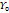- 2.5715 (1.0164) - 3.3879 (1.2586)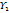- -0.5682 (0.2302) - -0.6109 (0.2796) Global Deviance 1237.203 1232.262 1235.81 1231.302 AIC: 1249.203 1246.262 1247.81 1245.302 BIC: 1271.641 1272.441 1270.249 1271.481

Table 2. Modeling results for salmonella hadar data.

 NB PIG Parameters Fixed Dispersion Parameter Varying Dispersion Parameter Fixed Dispersion Parameter Varying Dispersion Parameter Nu (ν) 1.0138 (0.0950) 0.9804 (0.1001) 1.0277 (0.0976) 0.9987 (0.1010) Beta (β) -0.0016 (0.0005) -0.0014 (0.0005) -0.0016 (0.0005) -0.0015 (0.0005) Gama (γ) -0.1426 (0.0643) -0.1409 (0.0639) -0.1481 (0.0654) -0.1449 (0.0650) Delta (δ) -0.4807 (0.0702) -0.4974 (0.0724) -0.4889 (0.0710) -0.5039 (0.0726) Lambda (λ) 0.0862 (0.0127) 0.0875 (0.0126) 0.0839 (0.0124) 0.0853 (0.0123) ln α; ln τ -1.500 (0.194) - -1.4009 (0.2092) -- 0.4871 (0.7636) - 0.5007 (0.7211)- -0.1782 (0.1729) - -0.1647 (0.1633) Global Deviance 1240.889 1239.903 1237.772 1236.804 AIC: 1252.889 1253.903 1249.772 1250.804 BIC: 1274.991 1279.688 1271.874 1276.589

Two conclusions can be obtained from the summary statistics within the Table 1 and 2. Foremost, both NB model and PIG model provided similar estimates. For instance, the Salmonella Agona data, both models showed that past observed cases of Salmonella Agona positively associated with the current infection frequency. This is as expected since the disease is infectious, hence exposure to it enhances its migration or transmission. Secondly, the PIG model showed better statistical fit for both datasets than the NB model when varying dispersion parameters were considered. This is the same case even for fixed dispersion parameter for both models. Models with varying dispersion parameters provided better statistical fit for the two datasets as compared to the models with fixed dispersion parameters. This is evidence that the Inverse Gaussian part of the PIG model is more flexible than the Gamma distribution in NB model in handling overdispersed datasets that are common for infectious diseases. This is enhanced by varying the dispersion parameter.

7.2. Residuals Analysis

True residuals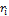, for any fitted model have a standard normal distribution if the fitted model is correct, irrespective of the model distribution. Table 3 and Table 4 give the summary of the randomized quantile residuals for the NB and PIG fitted models for salmonella hadar and salmonella agona datasets, respectively.

Table 3. Randomized quantile residuals summary for salmonella agona data.

 Mean Variance Skewness Kurtosis NB -0.007 1.02 0.15 3.00 PIG -0.009 1.05 -0.14 3.45

Table 4. Randomized quantile residuals summary for salmonella hadar data.

 Mean Variance Skewness Kurtosis NB 0.005 0.94 -0.004 3.88 PIG 0.01 0.95 0.23 3.59

The residuals summary of both the NB and PIG models for the salmonella hadar data behave well (their mean is nearly zero, variance nearly one, coefficient of skewness near zero, and the residuals values fall inside the "acceptance" region) except for the kurtosis for both the fitted models and skewness for PIG model. The residuals distribution kurtosis for both models is leptokurtic and the PIG model residuals distribution is rightly skewed.

For the salmonella agona data, the residuals summary of the PIG model suggests that the distribution of the residuals is leptokurtic and that from the NB suggest that the distribution is slightly skewed to the right.

Figure 3 and 4provide residual worm plots for both NB and PIG models for the salmonella hadar data respectively, while Figure 5 and 6 provide residual worm plots for both NB and PIG models for the salmonella agona data respectively.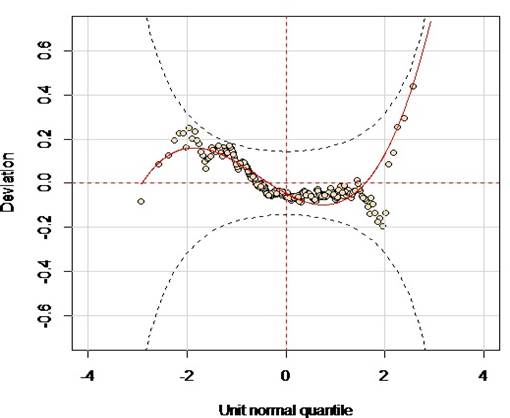Figure 3. Residuals worm plot for NB.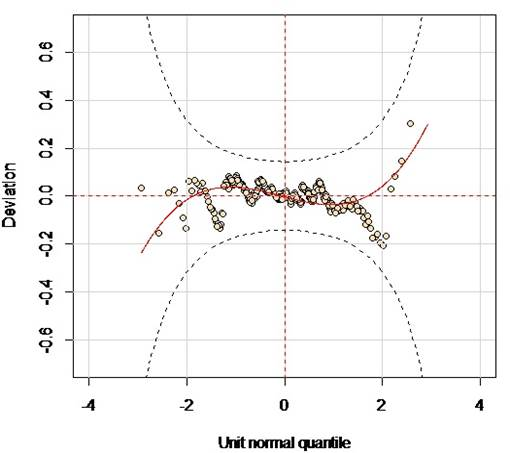Figure 4. Residuals worm plot for PIG.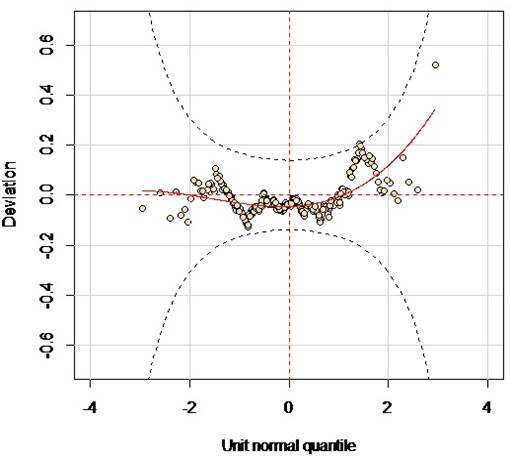Figure 5. Residuals worm plot for NB.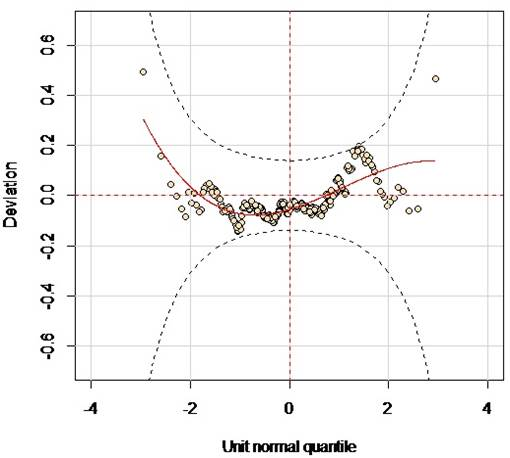Figure 6. Residuals worm plot for PIG.

Generally, the four residuals worm plots from the fitted NB and PIG models for both the salmonella agona and salmonella hadar data, indicate that both the NB model and PIG model fits the data well. A much deeper insight into the worm plots reveals some misfits of the cubic polynomial curve fitted to the residuals points for both the models considering the respective datasets. This explains the disparity shown from the summary of the randomized quantile residuals from both the fitted models for both the datasets. For the salmonella hadar data, the misfits are as follows; (-0.5, 3.5) and (5.5, 21.5) for the constant coefficient, (3.5, 5.5) for the linear coefficient, (-0.5, 3.5) and (5.5, 21.5) for the quadratic coefficient, and (1.5, 5.5) for the cubic coefficient of the cubic polynomial curve fitted to the residuals points. For the salmonella agona data, the misfits are as follows; (-0.5, 3.5) for the constant coefficient, (1.5, 5.5) for the linear coefficient, (-0.5, 3.5) and (5.5, 21.5) for the quadratic coefficient, and (1.5, 5.5) for the cubic coefficient of the cubic polynomial curve fitted to the residuals points.

7.3. Comparison of Prediction Performance

The analysis was done in two step approach. First, 80% of the entire datasets respectively were randomly selected from each dataset for model estimation. Based on this, a regression model was fitted. The fitted model was used for prediction purposes for the remaining 20% of the data sets respectively. A note to consider is that the model fitted for prediction performance was based on models with varying dispersion parameters since previously they provided a good statistical fit to the respective datasets.

Comparison of prediction accuracy for both the fitted NB model and PIG model was based on the Mean Absolute deviance (MAD). A better prediction is indicated by small values of MAD.

Table 5 gives a summary of modeling results of both the NB model and the PIG model.

Table 5. Modeling Results.

 NB PIG Parameters Salmonella Agona Salmonella Hadar Salmonella Agona Salmonella Hadar Nu (ν) 0.8939 (0.1314) 1.4244 (0.1251) 0.9068 (0.1384) 1.4240 (0.1257) Beta (β) -0.0015 (0.0007) -0.0039 (0.0007) -0.0016 (0.0008) -0.0039 (0.0007) Gama (γ) -0.4751 (0.0785) -0.2422 (0.0671) -0.4781 (0.0794) -0.2416 (0.0680) Delta (δ) -0.2409 (0.0734) -0.4763 (0.0731) -0.2510 (0.0737) -0.4757 (0.0734) Lambda (λ) 0.0731 (0.0178) 0.0335 (0.0163) 0.0730 (0.0180) 0.0348 (0.0163)3.3511 (1.0893) 0.4049 (0.9189) 6.4360 (1.4522) 0.3838 (0.8641)-0.6519 (0.2609) -0.2253 (0.2324) -0.7790 (0.3377) -0.1893 (0.2158) AIC: 982.2296 1034.436 981.1035 1031.829 MAD 1.2548 1.3604 1.2736 1.3721

The MAD values for both the NB model and PIG model are considerably almost the same, which therefore suggested that the PIG model can do as well as the NB model when it comes to prediction performance.

8. Conclusions and Recommendations

The aim of this study was to apply the PIG distribution in analyzing infectious disease count data. From the study, the PIG model provided better statistical fit than the traditional NB model. The statistical fit was further improved by varying the dispersion parameter of the respective models. These results can be attributed to the flexibility of the inverse Gaussian part of the PIG distribution as compared to the gamma part of the NB model. Hence, it can be said that PIG model can be an alternative model for analyzing infectious disease count data that exhibit overdispersion.

The applicability of the PIG model over NB model for analyzing infectious disease count data is not fully investigated considering that the datasets used in this study had small proportions of zero counts. The salmonella agona dataset had16% while salmonella hadar had 14%. Furthermore, the datasets were not that highly dispersed to better investigate the flexibility of the inverse Gaussian part of the PIG model. To cement the claim that PIG distribution can be a suitable alternative distribution to NB distribution in handling infectious disease count data, a simulation study should be performed.

References

1. Agresti A. (2002) Categorical Data Analysis. 2nd edition Wiley-Interscience.
2. Bulmer, M. G. 1979. Principles of statistics, New York, Dover Publications.
3. Cameron, A. C. &Trivedi, P. K. (1998). Regression Analysis of Count Data, New York, Cambridge University Press.
4. Dean, C., Lawless, J. F. &Willmot, G. E. (1989). A Mixed Poisson–Inverse-Gaussian Regression Model. Canadian Journal of Statistics, 17, 171-181.
5. Geedipally, S. R., Lord, D. &Dhavala, S. S. (2012). The negative binomial-Lindley generalized linear model: Characteristics and application using crash data. Accident Analysis & Prevention, 45, 258-265.
6. Gupta, R., Marino, B. S., Cnota, J. F., & Ittenbach, R. F. (2013). Finding the right distribution for highly skewed zero-inflated clinical data. Epidemiology Biostatistics and Public Health, 10 (1). DOI: 10.2427/8732.
7. Hauer E. (1997). Observational Before–after Studies in Road Safety: Estimating the Effect of Highway and Traffic Engineering Measures on Road Safety. ElsevierScience Ltd., Oxford, UK.
8. Held, L., Höhle, M., and Hofmann, M. (2005). A Statistical Framework for the analysis of Multivariate Infectious Disease Surveillance Counts. Statistical Modeling, 5:187-199.
9. Hofmann, M., (2006) Statistical Models for Infectious Disease Surveillance Counts.
10. Hu, M.-C., Pavlicova, M., &Nunes, E. V. (2011). Zero-inflated and Hurdle Models of Count Data with Extra Zeros: Examples from an HIV-Risk Reduction Intervention Trial. The American Journal of Drug and Alcohol Abuse, 37 (5),367–375.
11. Jagger, T. H. & Elsner, J. B. (2012). Hurricane Clusters in the Vicinity of Florida. Journal of Applied Meteorology and Climatology, 51, 869-877.
12. J.O. Lloyd-Smith, (2007). Maximum Likelihood estimation of the Negative Binomial dispersion parameter for highly overdispersed data, with application to infectious diseases. PLoS ONE.
13. Lee, J.-H., Han, G., Fulp, W. J., &Giuliano, A. R. (2012). Analysis of overdispersed count data: application to the Human Papilloma virus Infection in Men (HIM) Study. Epidemiology and Infection, 140 (6), 1087–1094.
14. Lord, D., Washington, S. P. & Ivan, J. N. (2005). Poisson, Poisson-Gamma and Zero-Inflated Regression Models of Motor Vehicle Crashes: Balancing Statistical Fit and Theory. Accident Analysis & Prevention, 37, 35-46.
15. Mullahy J. (1986). Specification and testing of some modified count data models. J Economy, 33:341–365.
16. Stasinopoulos, M. & Rigby, B. (2012). Generalized Additive Models for Location Scale and Shape.
17. Winkelmann R. (2008). Econometric Analysis of Count Data. 5th edition Springer;Berlin.
18. Zamani, H., Ismail, N., (2010). Negative binomial-Lindley distribution and Its application. Journal of Mathematics and Statistics 6 (1), 4-9.
19. Zha, L., Lord, D., &Zou, Y. (2014). The Poisson Inverse Gaussian (PIG) Generalized Linear Regression Model for Analyzing Motor Vehicle Crash Data. Submitted for publication.
20. Zhou, M., Li, L., Dunson, D., &Carin, L. (2012). Lognormal and Gamma Mixed Negative Binomial Regression. Proceedings of the... International Conference on Machine Learning. International Conference on Machine Learning, 2012, 1343–1350.
21. Zou, Y., Lord, D., Zhang, Y. &Peng, Y. (2013). Comparison of Sichel and Negative Binomial Models in Estimating Empirical Bayes Estimates. Transportation Research Board 92nd Annual Meeting.

 Contents 1. 2. 3. 4. 4.1. 4.2. 4.3. 4.4. 4.5. 5. 6. 6.1. 6.2. 7. 7.1. 7.2. 7.3. 8.
Article ToolsAbstractPDF(878K)